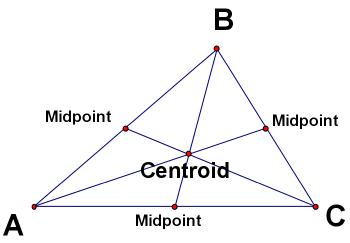index: click on a letter A B C D E F G H I J K L M N O P Q R S T U V W X Y Z A to Z index index: subject areas numbers & symbols sets, logic, proofs geometry algebra trigonometry advanced algebra & pre-calculus calculus advanced topics probability & statistics real world applications multimedia entrieswww.mathwords.com about mathwords website feedback

 Centroid For a triangle, this is the point at which the three medians intersect. In general, the centroid is the center of mass of a figure of uniform (constant) density.Centroid of a Triangle (Click on the image or here to launch an interactive java applet)     See also